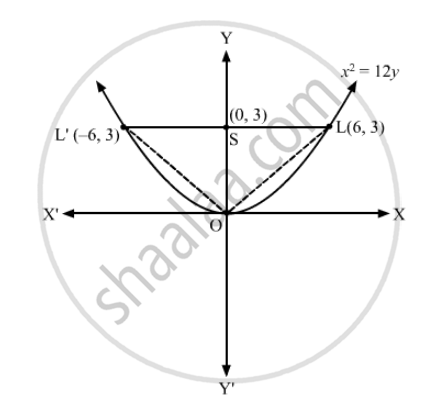Advertisement Remove all ads

# Find the area of the triangle formed by the lines joining the vertex of the parabola x 2 = 12 y to the ends of its latus rectum. - Mathematics

Find the area of the triangle formed by the lines joining the vertex of the parabola $x^2 = 12y$  to the ends of its latus rectum.

Advertisement Remove all ads

#### Solution

The given equation of the parabola is x2 = 12y.

On comparing the given equation with$x^2 = 4ay$

a = 3Required area = $\frac{1}{2}\left( LL' \times OS \right) = \frac{1}{2} \times 12 \times 3 = 18 \text{ square units }$

Is there an error in this question or solution?
Advertisement Remove all ads

#### APPEARS IN

RD Sharma Class 11 Mathematics Textbook
Chapter 25 Parabola
Exercise 25.1 | Q 6 | Page 25
Advertisement Remove all ads

#### Video TutorialsVIEW ALL 

Advertisement Remove all ads
Share
Notifications

View all notifications

Forgot password?